Author: Oscar Cronquist Article last updated on March 29, 2021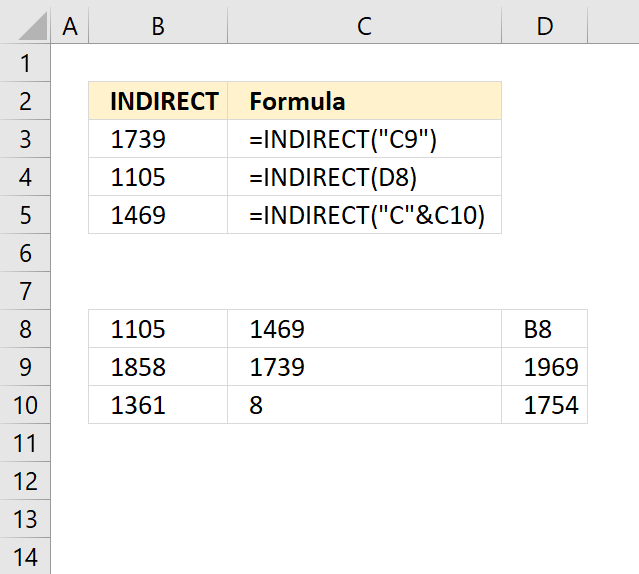The INDIRECT function returns the cell reference based on a text string and shows the content of that cell reference.

### Excel Function Syntax

INDIRECT(ref_text, [a1])

### Arguments

 ref_text Required. A reference to a cell, a name defined as a reference, or a reference to a cell as a text string. [a1] Optional. TRUE is the default value and is evaluated to A1- style reference. FALSE represents R1C1-style reference.
INDIRECT is a volatile function and extended use may slow your workbook considerably. Use with caution.
A volatile function recalculates more often than non-volatile Excel functions, this may cause heavy CPU-usage.
Other volatile Excel functions are: OFFSET | TODAY | NOW among others.
Conditional Formatting is super-volatile, it even recalculates if you are scrolling through data.

## 1. How to create a cell reference using the INDIRECT function?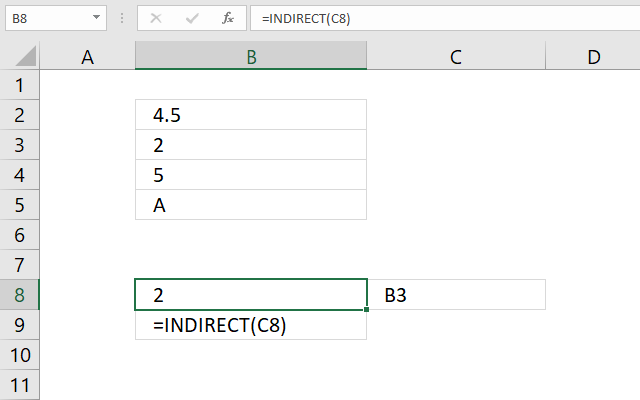The image above demonstrates a formula in cell B8 that uses a value in cell C8 to create a cell reference.

Formula in cell B8:

=INDIRECT(C8)

### 1.1 Explaining formula in cell B8

C8 returns "B3"

#### Step 2 - Create cell reference

INDIRECT(C8)

becomes

INDIRECT("B3")

and returns the value from cell B3 which is 2. See image above.## 2. How to use a worksheet name in the INDIRECT function?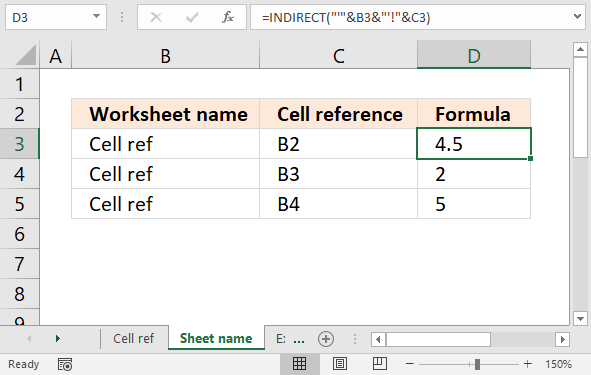The image above shows a formula in cell D3 that returns a value based on a worksheet name specified in cell B3 and a cell reference specified in cell C3.

Formula in cell D3:

=INDIRECT("'"&B3&"'"&"!"&C3)

### 2.1 Explaining formula in cell D3

#### Step 1 - Add quotes before and after worksheet name

The quotes are needed if there is a space character in the worksheet name.

"'"&B3&"'"

becomes

"'"&"Cell ref"&"'"

and returns

'Cell ref'

#### Step 2 - Add exclamation mark

The exclamation mark is used between the worksheet name and the cell reference.

"'"&B3&"'"&"!"

becomes

'Cell ref'&"!"

and returns

'Cell ref'!

#### Step 3 - Add cell reference

The cell reference i sin cell C3.

"'"&B3&"'"&"!"&C3

becomes

'Cell ref'!&C3

and returns

'Cell ref'!B2

#### Step 4 - Return value

INDIRECT("'"&B3&"'"&"!"&C3)

becomes

INDIRECT('Cell ref'!B2)

and returns the value from cell B2 in worksheet Cell ref.

## 3. How to VLOOKUP using the INDIRECT function?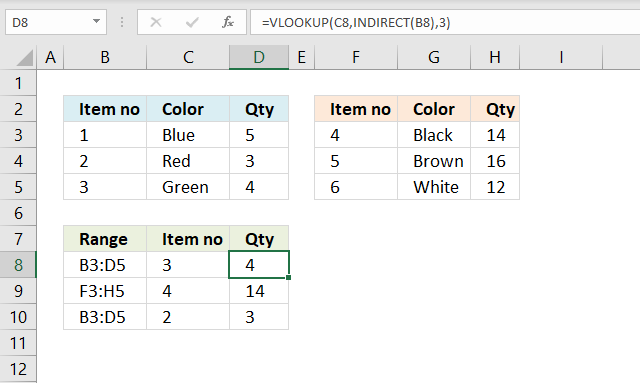The formula in cell D8, shown in the image above, contains a VLOOKUP function that uses the cell reference specified in cell B8 and a lookup value specified in cell C8 to return the correct value.

Formula in cell D8:

=VLOOKUP(C8, INDIRECT(B8), 3)

### 3.1 Explaining formula in cell D8

#### Step 1 - Create cell reference

INDIRECT(B8)

becomes

INDIRECT("B3:D5")

and returns

\$B\$3:\$D\$5

#### Step 2 - Evaluate VLOOKUP function

VLOOKUP(C8, INDIRECT(B8), 3)

becomes

VLOOKUP(C8, \$B\$3:\$D\$5, 3)

becomes

VLOOKUP(3, {1, "Blue", 5; 2, "Red", 3; 3, "Green", 4}, 3)

and returns 4.

## 4. How to use a range in the INDIRECT function?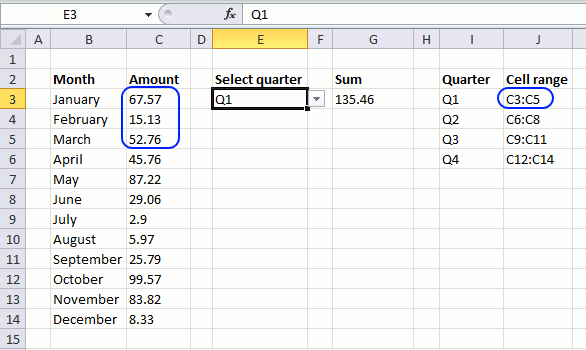The drop-down list in cell E3 lets you select a quarter, see the animated image above. The formula in cell G3 sums the corresponding values.

Formula in cell G3:

=SUM(INDIRECT(INDEX(\$J\$3:\$J\$6,MATCH(E3,I3:I6,0))))

### 4.1 Explaining formula in cell

#### Step 1 - Find relative position

The MATCH function returns the relative position of an item in an array or cell range that matches a specified value in a specific order.

MATCH(E3,I3:I6,0)

becomes

MATCH("Q1", {"Q1";"Q2";"Q3";"Q4"},0)

and returns 1.

#### Step 2 - Get value

The INDEX function returns a value from a cell range, you specify which value based on a row and column number.

INDEX(\$J\$3:\$J\$6, MATCH(E3, I3:I6,0))

becomes

INDEX(\$J\$3:\$J\$6, 1)

becomes

INDEX({"C3:C5"; "C6:C8"; "C9:C11"; "C12:C14"}, 1)

and returns "C3:C5".

#### Step 3 - Create cell reference

INDIRECT(INDEX(\$J\$3:\$J\$6, MATCH(E3, I3:I6,0)))

becomes

INDIRECT("C3:C5")

and returns {67.57; 15.13; 52.76}

#### Step 4 - Sum values

The SUM function adds numbers and returns a total.

SUM(INDIRECT(INDEX(\$J\$3:\$J\$6, MATCH(E3, I3:I6, 0))))

becomes

SUM({67.57; 15.13; 52.76})

and returns 135.46.

## 5. INDIRECT function - Address?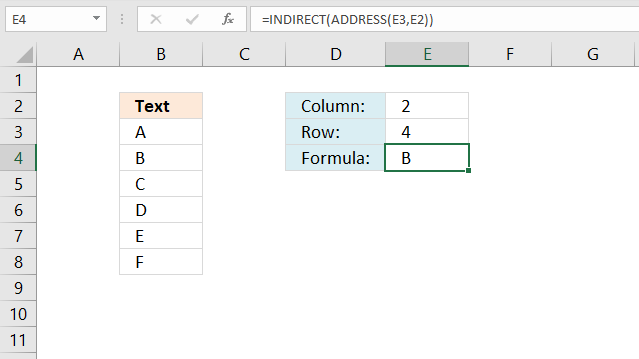The formula in cell E4 gets the column number from cell E2 and the row number from E3 and creates an address to a cell.

Formula in cell E4:

### 5.1 Explaining formula in cell

#### Step 1 - Create cell address

The ADDRESS function returns the address of a specific cell, you need to provide a row and column number.

becomes

and returns \$B\$4

#### Step 2 - Get value from cell

becomes

INDIRECT("\$B\$4")

and returns "B" to cell E4.

## 6. How to use the INDIRECT function to another workbook?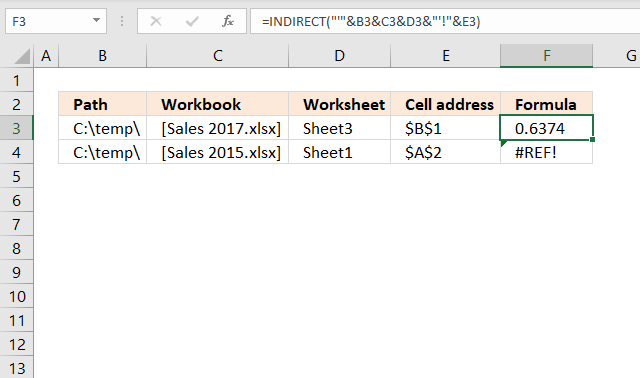The formula in cell E4 gets the column number from cell E2 and the row number from E3 and creates an address to a cell.

Formula in cell F3:

=INDIRECT("'"&B3&C3&D3&"'!"&E3)

### 6.1 Explaining formula in cell

#### Step 1 - Concatenate path, workbook name and worksheet

The ampersand character lets you concatenate values.

B3&C3&D3

becomes

"C:\temp\"&"[Sales 2017.xlsx]"&"Sheet3"

and returns

"C:\temp\[Sales 2017.xlsx]Sheet3"

#### Step 2 - Concatenate exclamation mark and single quotes

"'"&B3&C3&D3&"'!"

becomes

"'"&"C:\temp\[Sales 2017.xlsx]Sheet3"&"'!"

and returns

"'C:\temp\[Sales 2017.xlsx]Sheet3'!"

#### Step 3 - Concatenate cell address

"'"&B3&C3&D3&"'!"&E3

becomes

"'C:\temp\[Sales 2017.xlsx]Sheet3'!"&E3

and returns

"'C:\temp\[Sales 2017.xlsx]Sheet3'!\$B\$1"

#### Step 4 - Create cell reference

INDIRECT("'"&B3&C3&D3&"'!"&E3)

becomes

INDIRECT("'C:\temp\[Sales 2017.xlsx]Sheet3'!\$B\$1")

and returns 0.6374.

## 7. How to create an INDIRECT sum?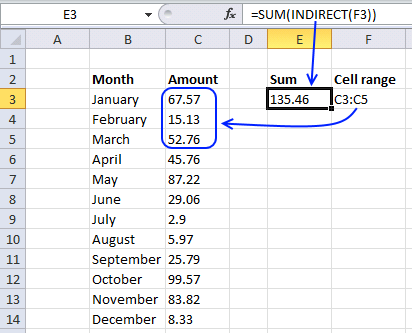The image above demonstrates a formula in cell E3 that uses the specified cell range in F3 to sum values.

Formula in cell E3:

=SUM(INDIRECT(F3))

Cell A1 contains C3:C5. Sum function sums values in cell C3:C5. If you change the value in cell F3 to C3:C6 it sums the values in cell range C3:C6.

## 8. How to use INDIRECT to a column number?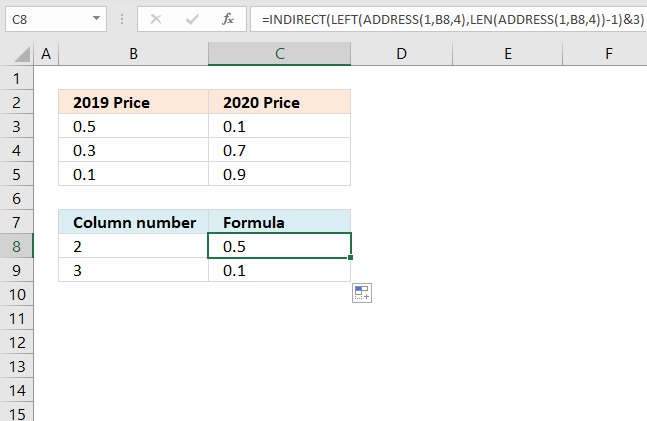The formula in cell C8 creates a cell reference based on the specified number in cell B8.

Formula in cell F3:

### 8.1 Explaining formula in cell C8

#### Step 1 - Create an address

The ADDRESS function ceturns the address of a specific cell, you need to provide a row and column number.

becomes

and returns "B1".

#### Step 2 - Calculate string length

The LEN function counts the number of characters in a given string.

becomes

LEN("B1")-1

becomes

2-1

and returns 1.

#### Step 3 - Extract column letter from address

The LEFT function extracts a specific number of characters always starting from the left.

LEFT(text, [num_chars])

becomes

LEFT("B1", 1)

and returns "B".

#### Step 4 - Create cell reference

becomes

INDIRECT("B"&3)

becomes

INDIRECT("B3")

and returns 0.5.

You can also use the R1C1 system to create a cell reference to a column number, see the next section below.

## 9. How to use the INDIRECT function with R1C1?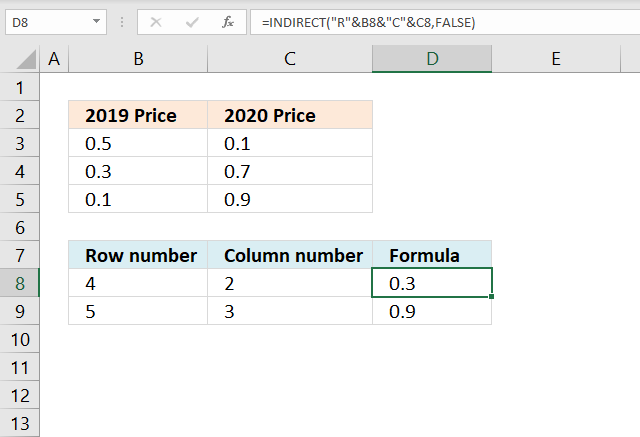The formula in cell D8

Formula in cell D8 :

=INDIRECT("R"&B8&"C"&C8,FALSE)

### 9.1 Explaining formula in cell C8

#### Step 1 - Concatenate strings

The ampersand character lets you concatenate values.

"R"&B8&"C"&C8

becomes

"R"&4&"C"&2

and returns R4C2.

#### Step 2 - Create cell reference

The INDIRECT function has the following arguments: INDIRECT(ref_text[a1])

[a1] stands for the style reference. TRUE is the default value and is evaluated to A1-style reference. FALSE represents R1C1-style reference.

INDIRECT("R"&B8&"C"&C8,FALSE)

becomes

INDIRECT("R4C2",FALSE)

and returns 0.3 or the value from cell B4. Cell B4 is the intersection between column 2 and row 4.

## 10. How to hardcode a cell reference?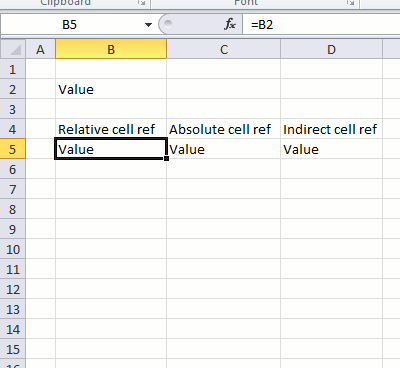When you insert or delete a row or column in excel the cell references in formulas change, even if you use absolute cell references.

The Indirect function helps you solve that problem. The animated picture above demonstrates what happens with relative, absolute, and indirect cell references when you insert a row.

### Get the Excel fileIndirect-functionv2.xlsx